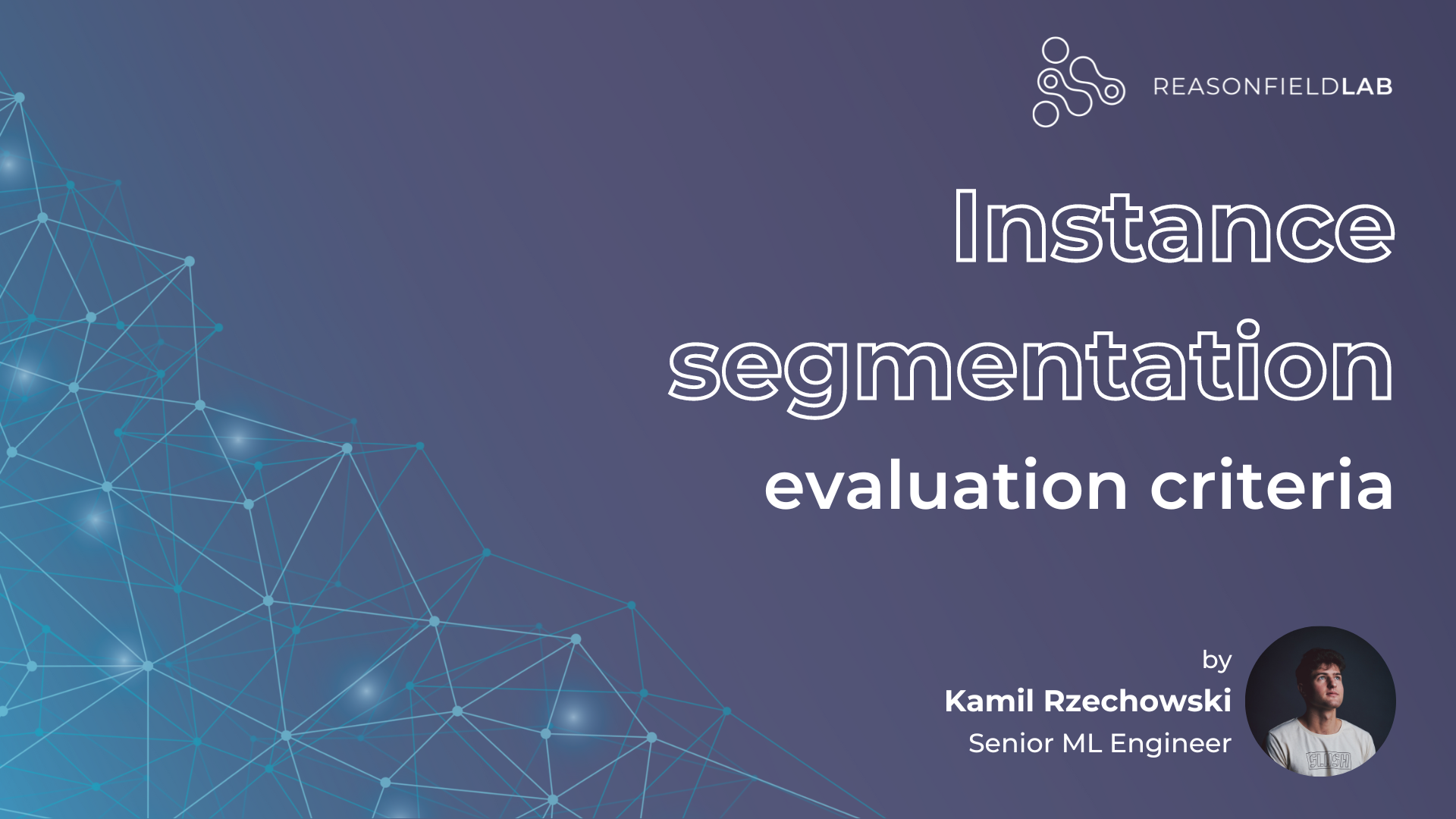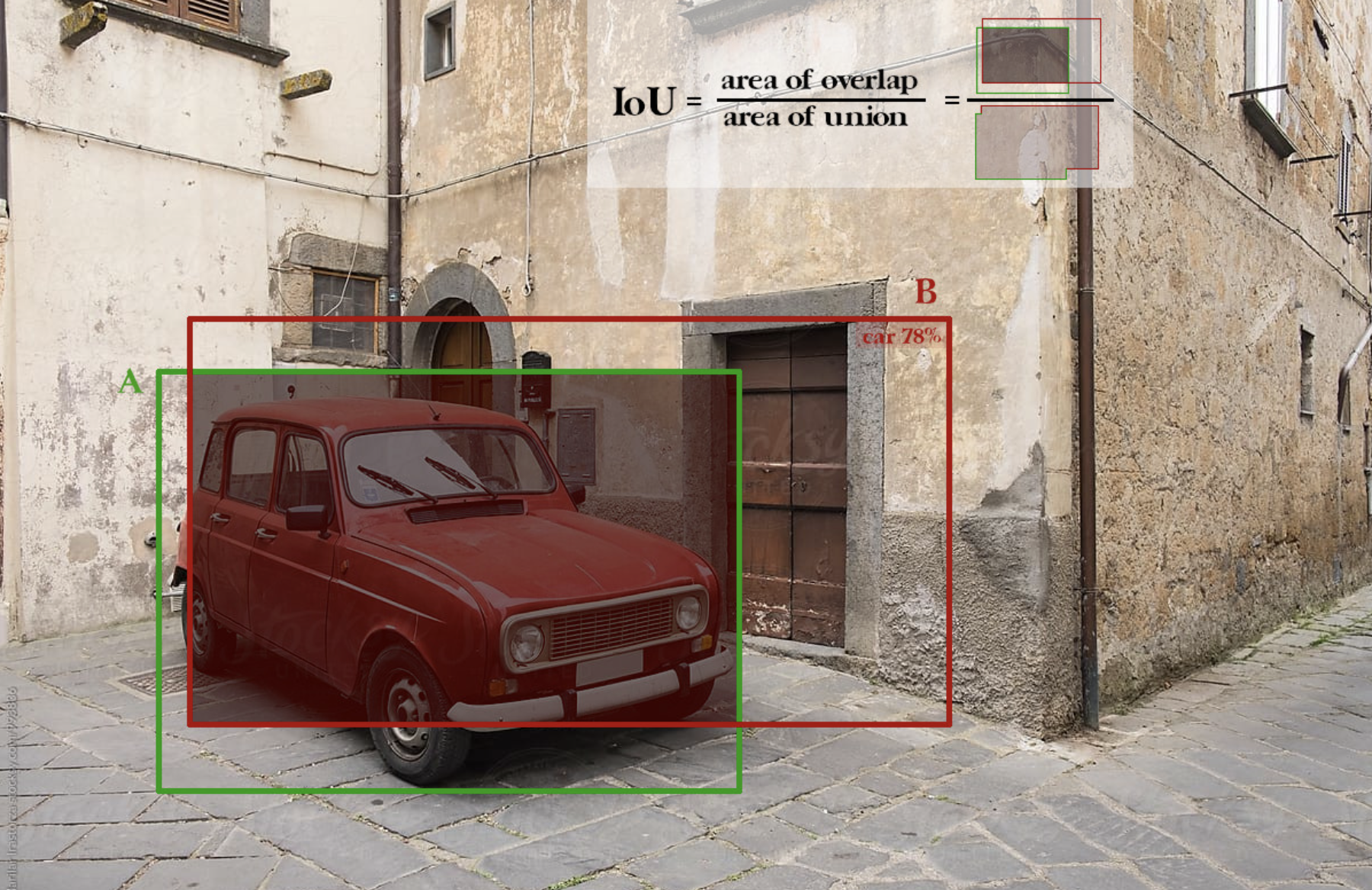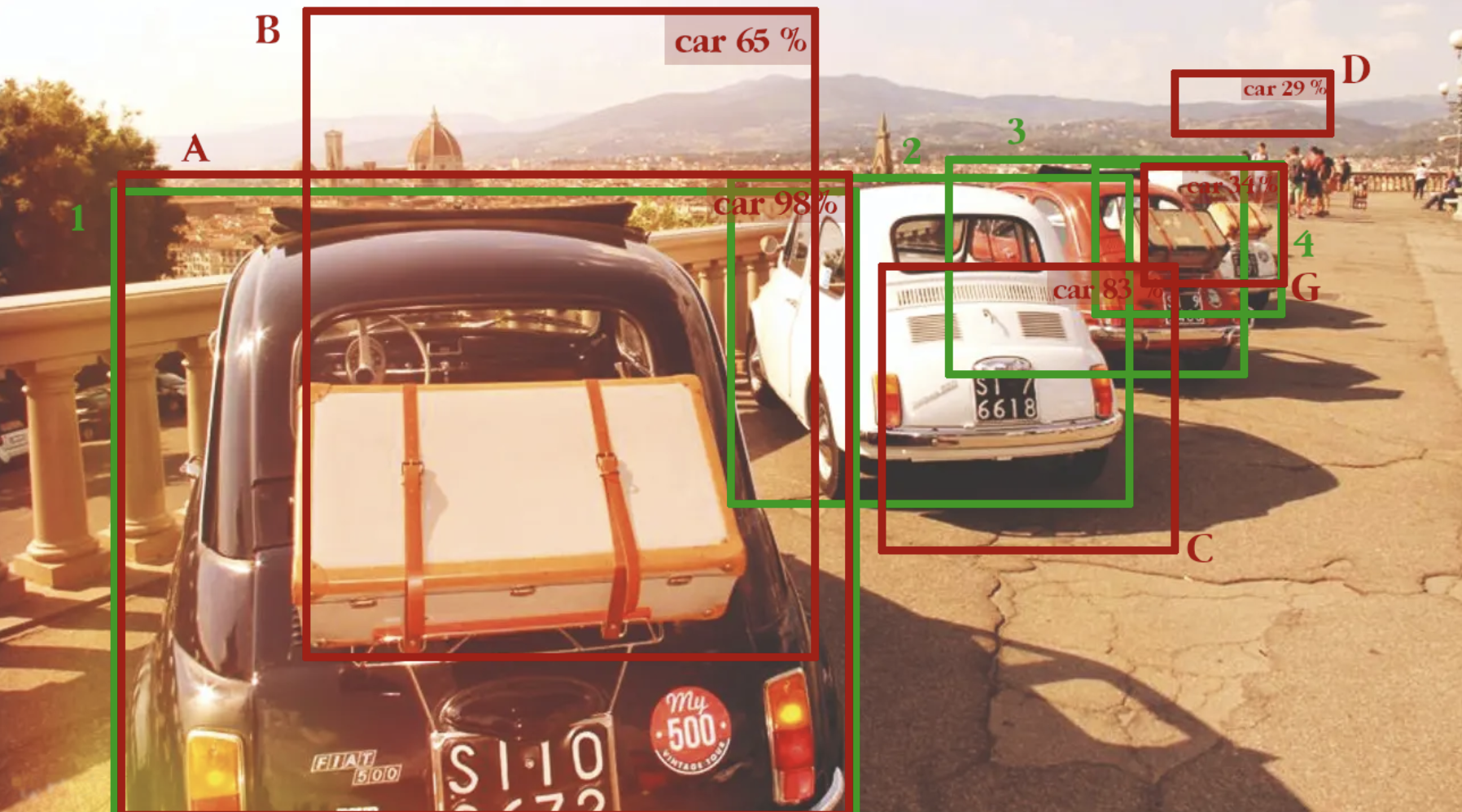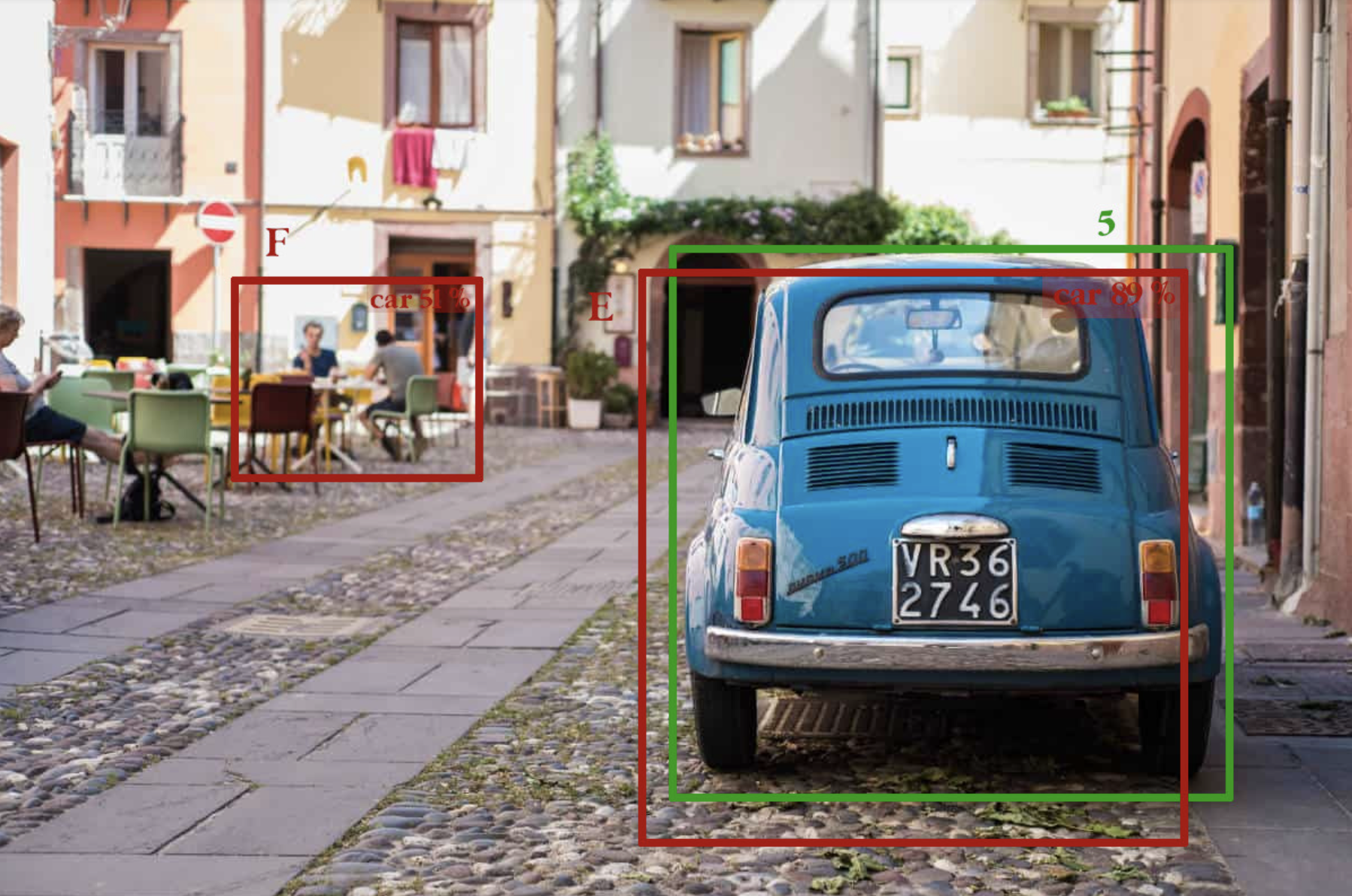August 16, 2022

# Instance segmentation evaluation criteria# Evaluating instance segmentation algorithms

Instance segmentation is an extension of object detection where we not only localize an object but also generate a binary mask for each single object detected. There are two main categories of instance segmentation algorithms: detection base instance segmentation algorithms and single-shot instance segmentation algorithms.

The first approach generates higher quality masks, however, the latter one is faster. My previous article, Instance segmentation algorithms overview, dives deep into types and approaches used to perform instance segmentation and the upcoming article (coming soon, stay tuned!) walks through loss functions used for instance segmentation model training. On the other hand, the following article focuses on metrics used to evaluate the instance segmentation algorithms. The most commonly used is mean Average Precision (mAP). But let’s start from the beginning.

## IoU

The basic evaluation criterion for measuring the quality of the generated masks is Intersection over Union (IoU). It measures the intersection area between two bounding boxes or, in the case of instance segmentation, it measures the intersection area between two masks. The idea of IoU is best explained by the image below.Figure 1: Green - ground truth, red - prediction. Source: own elaboration based on image acquired from Stocksy

The maximum possible IoU value is 1 and it means that the predicted and ground truth masks perfectly overlap each other. On the other hand, in the worst-case scenario, the IoU has a value of 0. It is worth mentioning that the IoU is equal to 0 in each situation when there is no overlap between ground truth and the predicted mask. So it doesn’t matter if two masks are close to each other or far away, if there is no overlap, the IoU will always be zero. For that reason, the IoU metric cannot be used for loss function as it is not fully differentiable. There is a variant of IoU called Generalized IoU that converges to zero as two masks move away from each other, however, it is never equal to 0. Generalized IoU is fully differentiable and thus can be used as a loss function for object detection and instance segmentation tasks.

In most cases, the IoU is used as an intermediate step for calculating the mean Average Precision (mAP). Using pure mIoU is impractical, as there is one more drawback. Let's imagine two instance segmentation networks. The first network is predicting very good masks (in terms of IoU) but always generates one mask per image, while usually, multiple objects are present in a single image. The second network predicts worse masks (in terms of IoU) but generates masks for every single object in the image. Taking into account only IoU, the first network would outperform the second network. However, the first network evaluation doesn’t consider objects the network didn’t predict. In the overall assessment, the second network should obtain a better score. It is overcome by the mAP evaluation metric.

### mAP

Mean average overlap uses the concept of Precision and Recall.

Let’s use image 1 and image 2 as an example to work on.Figure 2: Example of bbox detection by an object detection algorithm. Red - predictions, green - ground truth. Source: own elaboration based on image acquired hereFigure 3: Example of bbox detection by an object detection algorithm. Red - predictions, green - ground truth. Source: own elaboration based on image acquired here

True Positives are masks that were correctly predicted (example bboxes A, C, G), False Negatives are masks that were not predicted (example bbox 3), False Positives are masks that were predicted but shouldn’t be predicted (example bboxes B, D). In order to classify if a mask (bbox) is TP or FP, we calculate IoU between a predicted mask and a ground truth mask and threshold by some value. Depending on the evaluation criteria, the threshold varies from 0.5 to 0.95. For example, if the IoU between the ground truth mask and the predicted mask is >= 0.5, then the predicted mask is classified as TP, otherwise as FP. If there are more than one detections for ground truth, the first detection is considered a TP and the rest as False Positive (example boxes A and B. A is TP and B is FP).

The Average Precision metric computes the Area Under the Curve (AUC) of the Precision-Recall curve. The higher the value, the better the instance segmentation algorithm. When only comparing the Precision-Recall curve, an instance segmentation algorithm is considered good if precision stays high as recall increases. The AUC is preferred over plot comparisons as it is a single value that can be easily compared between models.

## How to calculate recall

In a recall calculation, the denominator is constant and is equal to the ground truth masks in the evaluation set. In the example above, the recall’s denominator is always equal to 5. In order to compute the Precision-Recall curve, the following steps are taken:

1. Sort all predictions by confidence
2. Go prediction by prediction and calculate the accumulated TP and accumulated FP. If the given prediction is FP, increase the Acc TP by one (TP += 1). Else, if the given prediction is FP, increase the FP by one (FP += 1). Then, compute Precision and Recall at the given stage with the Precision and Recall formulas.
3. Use all computed Precision and Recall values to plot the Precision-Recall curve.

The Mean Average Overlap measures the mean value of AP for IoU from 0.5 to 0.95 with a 0.05 step interval. For COCO evaluation, AP is equal to mAP.

Mean Average Overlap calculation example

The mean Average Overlap for IoU > 0.5.

The Precision-Recall curve follows a zigzag pattern. To calculate the AUC of the curve, the 11-points interpolation method is used in most object detection and instance segmentation challenges (PASCAL VOS, COCO). Precision is averaged in the set of 11 equally spaced Recall levels [0.0, 0.1, 0.2 …. 1.0].

where p(r) - is the precision at the given recall level.

Very good mAP computation example with explanation and code can be found here.

Below is a list of all IoU thresholds used by the COCO instance segmentation metric.

## Summary

The mAP is the most commonly used metric for object detection and segmentation algorithms. It penalizes miss detections and it measures the mask quality well. Moreover, it is just a single value, which can be easily compared between models. The mAP builds on top of IoU and the precision-recall curve. Based on the IoU threshold it classifies predictions as TP or FP. Further based on obtained TP and FP values it computes the precision-recall curve. It uses the PR AUC to obtain a single value. Depending on the challenge different IoU thresholds are chosen, but usually, the threshold varies between 0.5 and 0.95.